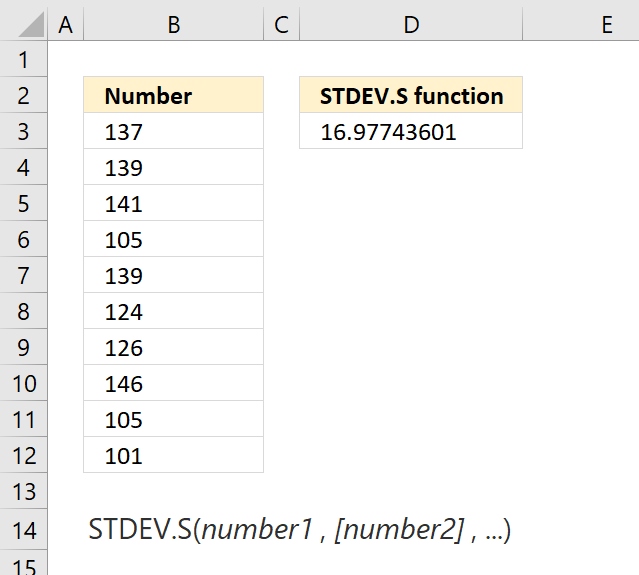Author: Oscar Cronquist Article last updated on June 15, 2018The STDEV.S function returns standard deviation based on a sample of the entire population.

The standard deviation is how widely numbers are distributed around the average value.

Formula in cell D3:

=STDEV.S(B3:B12)

### Excel Function Syntax

STDEV.S(number1, [number2], ...)

### Arguments

 number1 Required. The first number argument that represents a sample of the population. [number2] Optional. Up to 253 additional number arguments.

### Comments

The STDEV.S function ignores logical values and text values.

For large sample sizes, STDEV.S and STDEV.P return approximately equal values.